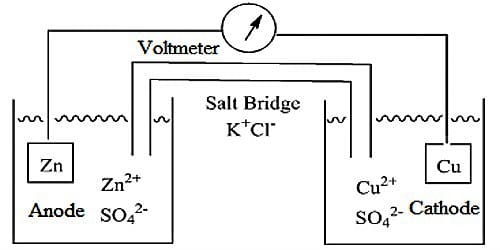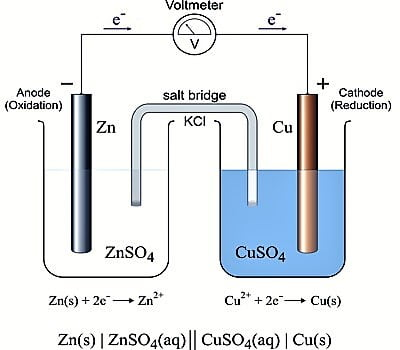# Reversible and Irreversible Cells

Reversible and Irreversible Cells

A Galvanic cell always has two electrodes in which there is a difference in the tendency to give up electrons. The electrodes are properly arranged so that current can flow. Galvanic cells may be either reversible or irreversible in the thermodynamic sense. A reversible cell is one which does not give any current when it is connected in an opposite sense to an outside source of e.m.f., exactly equal to that of the cell. If the e.m.f. of the external source is infinitesimally higher than that of the cell then the current will go in the opposite direction, i.e., the cell reaction will be reversed; on the other hand, if the value is slightly lower current will flow from the cell. Daniel cell is an example of a reversible cell.

The chemical reaction of the cell is reversed and the current flows in the opposite direction when the external emf is slightly higher than that of the cell. Any other cell, which does not obey the above two conditions, is termed as irreversible. Daniel cell is reversible but Zn, H2SO4, Ag, etc., the cell is irreversible in nature

When in a cell reaction gas is evoked or a precipitate is formed the cell is irreversible because the cell cannot be brought back to its original condition by reversing the current. As an example of an irreversible cell consider zinc and copper electrodes which dip into a solution of sulphuric acid. When the cell is producing current the cell reaction will be

Zn (s) + 2 H+ (aq) ↔ Zn2+ (aq) + H2 (g)

The zinc electrode dissolves to form zinc ions, and hydrogen is liberated at the copper electrode. When the current is reversed the above reaction is not reversed. Instead, the following reaction takes place-

Cu (s) + 2 H+ (aq) ↔ Cu2+ (aq) + H2 (g)

Copper goes into solution and hydrogen gas is liberated.

Irreversible cells are those which necessitate alternate of chemicals when they give out electricity these cannot be recharged.

Irreversible cells are not amenable to exact theoretical treatment. For reversible cells, thermodynamic principles may be applied. The work done in a reversible cell is the maximum and the maximum work can be related to other thermodynamic quantities. In reversible cells, both the electrodes are reversible with respect to one or other of the ions in solution.Reversible electrochemical cells are the cells whose cell reactions can be get reversed when an external emf better than its capability is applied. (A cell which obeys thermodynamic conditions of reversibility is known as reversible cells). For example Daniel cell with Capacity 1.1 V, when an external emf of 1.1 V is applied, the cell reaction stops.

Zn + Cu+2 → Zn+2 + Cu.

But when an increased amount of emf better than 1.1 V is applied, the cell reaction is get reversed.

Zn+2 + Cu → Zn + Cu+2

A cell of this type can be termed as Reversible cell.

A cell is said to be reversible if the following two conditions are fulfilled –

1. The chemical reaction of the cell stops when an accurately equal external emf is applied.
2. The chemical reaction of the cell is reversed and the current flows in a reverse course when the external emf is somewhat higher than that of the cell.

Cells produce electricity when electrochemical reactions take place in the cell. If the cell is reversible (like a lead-acid car battery, or a nickel cadmium battery) you can use an upper voltage to force current backward through the battery, causing the reverse electrochemical reactions to occur, recharging the battery. A reversible cell can reach the chemical and physical requirements of stability and the chemical effect will reverse with energy input.